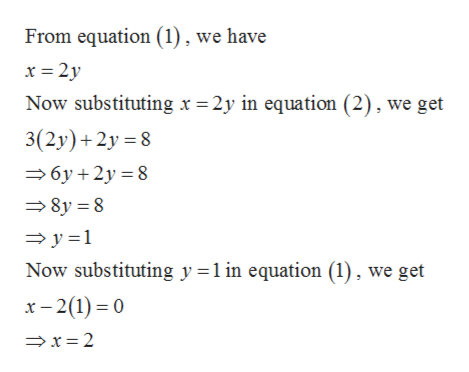# x-2y=03x+2y=8solve by the method of substitution

Question
57 views

x-2y=0

3x+2y=8

solve by the method of substitution

check_circle

Step 1

Given,

Step 2

Solving the equations...help_outlineImage TranscriptioncloseFrom equation (1), we have x 2y Now substituting x =2y in equation (2), we get 3(2y) 2y 8 6y 2y8 8y 8 y 1 Now substituting y l in equation (1), we get x-2(1)0 x 2 fullscreen

### Want to see the full answer?

See Solution

#### Want to see this answer and more?

Solutions are written by subject experts who are available 24/7. Questions are typically answered within 1 hour.*

See Solution
*Response times may vary by subject and question.
Tagged in

### Equations and In-equations